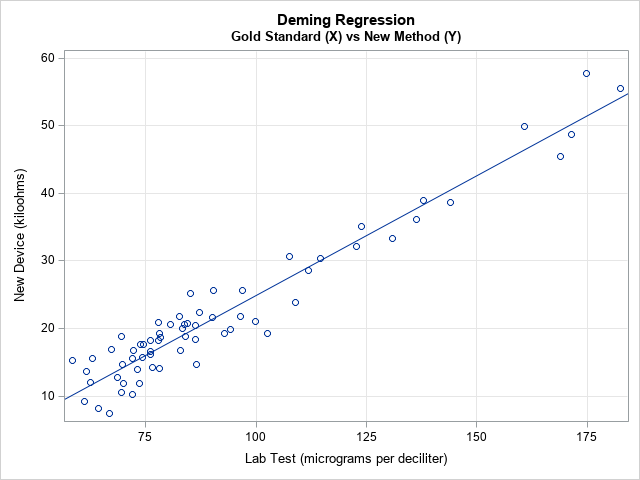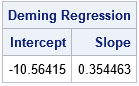Deming regression (also called errors-in-variables regression) is a total regression method that fits a regression line when the measurements of both the explanatory variable (X) and the response variable (Y) are assumed to be subject to normally distributed errors. Recall that in ordinary least squares regression, the explanatory variable (X) is assumed to be measured without error. Deming regression is explained in a Wikipedia article and in a paper by K. Linnet (1993).

A situation in which both X and Y are measured with errors arises when comparing measurements from different instruments or medical devices. For example, suppose a lab test measures the amount of some substance in a patient's blood. If you want to monitor this substance at regular intervals (for example, hourly), it is expensive, painful, and inconvenient to take the patient's blood multiple times. If someone invents a medical device that goes on the patient's finger and measures the substance indirectly (perhaps by measuring an electrical property such as bioimpedance), then that device would be an improved way to monitor the patient. However, as explained in Deal, Pate, and El Rouby (2009), the FDA would first need to approve the device and determine that it measures the response as accurately as the existing lab test. The FDA encourages the use of Deming regression for method-comparison studies.

### Deming regression in SAS

There are several ways to compute a Deming Regression in SAS. The SAS FASTats site suggests maximum likelihood estimation (MLE) by using PROC OPTMODEL, PROC IML, or PROC NLMIXED. However, you can solve the MLE equations explicitly to obtain an explicit formula for the regression estimates. Deal, Pate, and El Rouby (2009) present a rather complicated macro, whereas Njoya and Hemyari (2017) use simple SQL statements. Both authors also provide SAS code for estimating the variance of the Deming regression estimates, either by using the jackknife method or by using the bootstrap. However, the resampling schemes in both papers are inefficient because they use a macro loop to perform the jackknife or bootstrap.

The following SAS DATA Step defines pairs of hypothetical measurements for 65 patients, each of whom received the standard lab test (measured in micrograms per deciliter) and the new noninvasive device (measured in kiloohms):

```data BloodTest; label x="micrograms per deciliter" y="kiloOhms"; input x y @@; datalines; 169.0 45.5 130.8 33.4 109.0 23.8 94.1 19.8 86.3 20.4 78.4 18.7 76.1 16.1 72.2 16.7 70.0 11.9 69.8 14.6 69.5 10.6 68.7 12.7 67.3 16.9 174.7 57.8 137.9 39.0 114.6 30.4 99.8 21.1 90.1 21.7 85.1 25.2 80.7 20.6 78.1 19.3 77.8 20.9 76.0 18.2 77.8 18.3 74.2 15.7 73.1 13.9 182.5 55.5 144.0 38.7 123.8 35.1 107.6 30.6 96.9 25.7 92.8 19.2 87.2 22.4 86.3 18.4 84.4 20.7 83.7 20.6 83.3 20.0 83.9 18.8 82.7 21.8 160.8 49.9 122.7 32.2 102.6 19.2 86.6 14.7 76.1 16.6 69.6 18.8 66.7 7.4 64.4 8.2 63.0 15.5 61.7 13.7 61.2 9.2 62.4 12.0 58.4 15.2 171.3 48.7 136.3 36.1 111.9 28.6 96.5 21.8 90.3 25.6 82.9 16.8 78.1 14.1 76.5 14.2 73.5 11.9 74.4 17.7 73.9 17.6 71.9 10.2 72.0 15.6 ;   title "Deming Regression"; title2 "Gold Standard (X) vs New Method (Y)"; proc sgplot data=BloodTest noautolegend; scatter x=x y=y; lineparm x=0 y=-10.56415 slope=0.354463 / clip; /* Deming regression estimates */ xaxis grid label="Lab Test (micrograms per deciliter)"; yaxis grid label="New Device (kiloohms)"; run;```The scatter plot shows the pairs of measurements for each patient. The linear pattern indicates that the new device is well calibrated with the standard lab test over a range of clinical values. The diagonal line represents the Deming regression estimate, which enables you to convert one measurement into another. For example, a lab test that reads 100 micrograms per deciliter is expected to correspond to 25 kiloohms on the new device and vice versa. (If you want to convert the new readings into the old, you can regress X onto Y and plot X on the vertical axis.)

The following SAS/IML function implements the explicit formulas that compute the slope and intercept of the Deming regression line:

```/* Deming Regression in SAS */ proc iml; start Deming(XY, lambda=); /* Equations from https://en.wikipedia.org/wiki/Deming_regression */ m = mean(XY); xMean = m; yMean = m; S = cov(XY); Sxx = S[1,1]; Sxy = S[1,2]; Syy = S[2,2]; /* if lambda is specified (eg, lambda=1), use it. Otherwise, estimate. */ if IsEmpty(lambda) then delta = Sxx / Syy; /* estimate of ratio of variance */ else delta = lambda; c = Syy - delta*Sxx; b1 = (c + sqrt(c**2 + 4*delta*Sxy**2)) / (2*Sxy); b0 = yMean - b1*xMean; return (b0 || b1); finish;   /* Test the program on the blood test data */ use BloodTest; read all var {x y} into XY; close; b = Deming(XY); print b[c={'Intercept' 'Slope'} L="Deming Regression"];```The SAS/IML function can estimate the ratio of the variances of the X and Y variable. In the SAS macros by Deal, Pate, and El Rouby (2009) and Njoya and Hemyari (2017), the ratio is a parameter that is determined by the user. The examples in both papers use a ratio of 1, which assumes that the devices have an equal accuracy and use the same units of measurement. In the current example, the lab test and the electrical device use different units. The ratio of the variances for these hypothetical devices is about 7.4.

### Standard errors of estimates

You might wonder how accurate the parameter estimates are. Linnet (1993) recommends using the jackknife method to answer that question. I have previously explained how to jackknife estimates in SAS/IML, and the following program is copied from that article:

```/* Helper modules for jackknife estimates of standard error and CI for parameters: */ /* return the vector {1,2,...,i-1, i+1,...,n}, which excludes the scalar value i */ start SeqExclude(n,i); if i=1 then return 2:n; if i=n then return 1:n-1; return (1:i-1) || (i+1:n); finish;   /* return the i_th jackknife sample for (n x p) matrix X */ start JackSamp(X,i); return X[ SeqExclude(nrow(X), i), ]; /* return data without i_th row */ finish;   /* 1. Compute T = statistic on original data */ T = b;   /* 2. Compute statistic on each leave-one-out jackknife sample */ n = nrow(XY); T_LOO = j(n,2,.); /* LOO = "Leave One Out" */ do i = 1 to n; J = JackSamp(XY,i); T_LOO[i,] = Deming(J); end;   /* 3. compute mean of the LOO statistics */ T_Avg = mean( T_LOO );   /* 4. Compute jackknife estimates of standard error and CI */ stdErrJack = sqrt( (n-1)/n * (T_LOO - T_Avg)[##,] ); alpha = 0.05; tinv = quantile("T", 1-alpha/2, n-2); /* use df=n-2 b/c both x and y are estimated */ Lower = T - tinv#stdErrJack; Upper = T + tinv#stdErrJack; result = T` || T_Avg` || stdErrJack` || Lower` || Upper`; print result[c={"Estimate" "Mean Jackknife Estimate" "Std Error" "Lower 95% CL" "Upper 95% CL"} r={'Intercept' 'Slope'}];```The formulas for the jackknife computation differs slightly from the SAS macro by Deal, Pate, and El Rouby (2009). Because both X and Y have errors, the t quantile must be computed by using n–2 degrees of freedom, not n–1.

If X and Y are measured on the same scale, then the methods are well-calibrated when the 95% confidence interval (CI) for the intercept includes 0 and the CI for the intercept includes 1. In this example, the devices use different scales. The Deming regression line enables you to convert from one measurement scale to the other; the small standard errors (narrow CIs) indicate that this conversion is accurate.

In summary, you can use a simple set of formulas to implement Deming regression in SAS. This article uses SAS/IML to implement the regression estimates and the jackknife estimate of the standard errors. You can also use the macros that are mentioned in the section "Deming regression in SAS," but the macros are less efficient, and you need to specify the ratio of the variances of the data vectors.

The post Deming regression for comparing different measurement methods appeared first on The DO Loop.